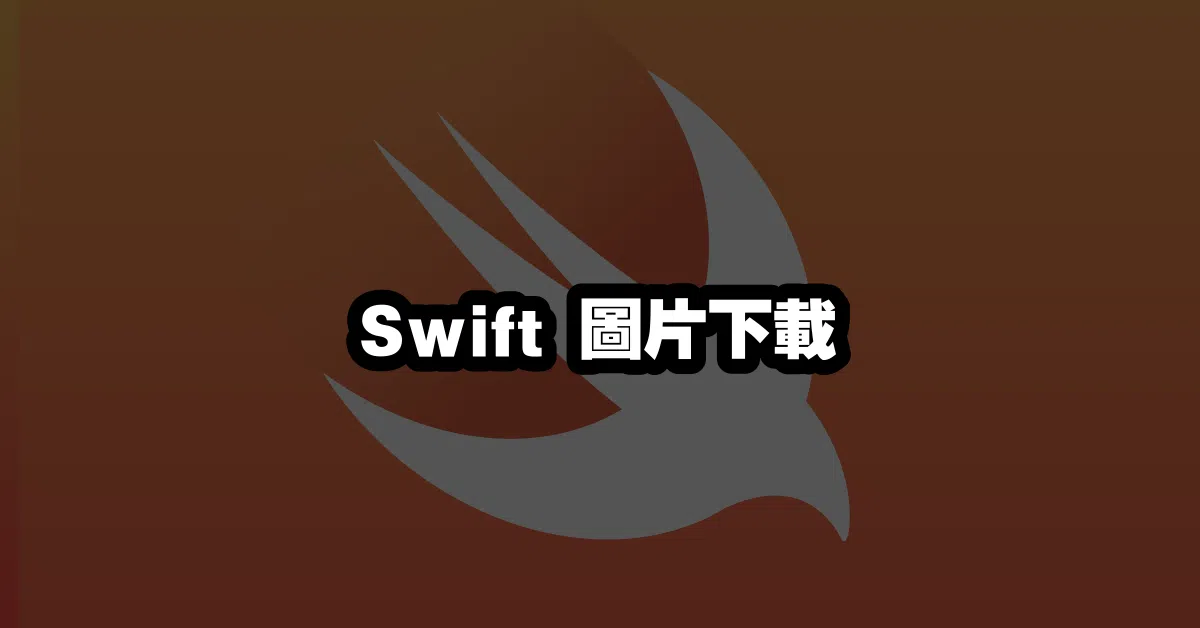# Swift 圖片下載 🖼️

Swift 是一種快速、安全的程式語言，可以用於開發 iOS、macOS、watchOS 和 tvOS 等應用程式。本文將介紹如何使用 Swift 來下載圖片。

## 使用 Swift 下載圖片

``````let session = URLSession.shared
``````

``````let url = URL(string: "https://example.com/image.jpg")!
``````

``````let task = session.dataTask(with: url) { data, response, error in
// Handle the response here
}
``````

`dataTask` 方法的閉包中，我們可以檢查 `data``error` 參數，以確保下載操作成功完成：

``````if let error = error {
// Handle the error
} else if let data = data {
// Handle the data
}
``````

``````let image = UIImage(data: data)
``````

``````task.resume()
``````

## 推薦閱讀文章

``````            <a href="https://www.raywenderlich.com/567-swift-image-downloading-and-caching-tutorial">Swift 圖片下載與快取教學</a><br>